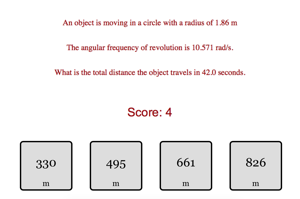Programs for Introduction to Circular Motion
Below are all the programs that might be used with this topic. Click on "See Resources" to see if there are any student directions, lab sheets or other materials that have been created for this program.
Force Direction Relative to Motion Lab

LabCircular Motion Learning Lab

Lab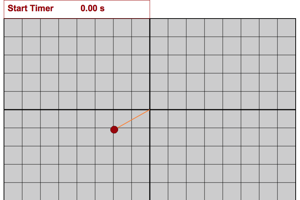Classic Circular Force

LabCircular Acceleration Lab

LabKinetic Energy of a Ball Moving in a Circle

Homework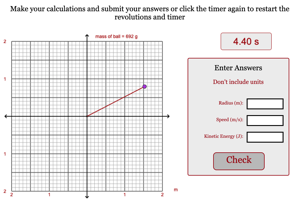Oh Snap!

HomeworkSlice the String

Game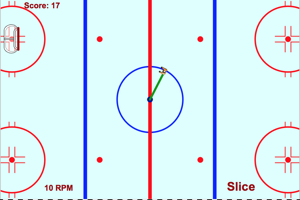Circular Motion Self Test

Game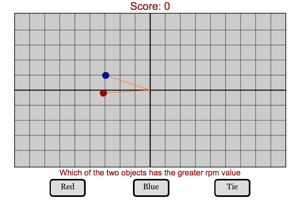Quick Circular Motion Calculation Challenge

Game